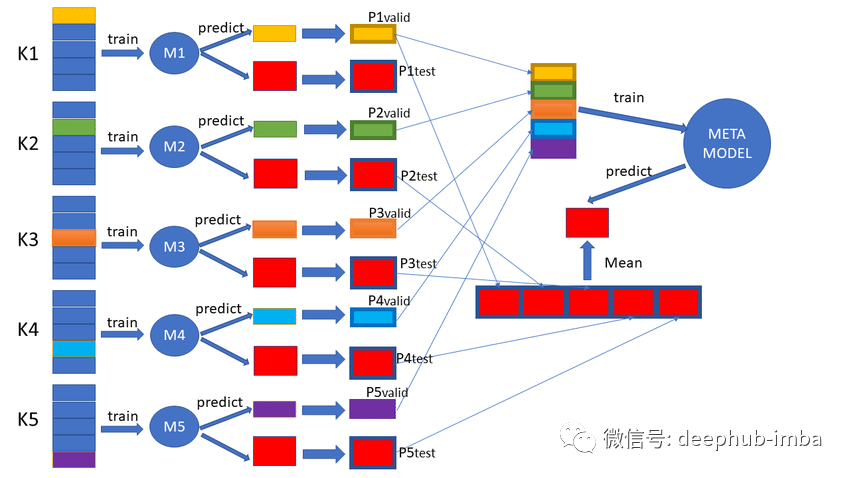1

# 使用折外预测（oof）评估模型的泛化性能和构建集成模型

• 折外预测是对不用于训练模型的数据进行的一种样本外预测。
• 在对看不见的数据进行预测时，折外预测最常用于估计模型的性能。
• 折外预测可用于构建集成模型，称为堆叠泛化或堆叠集成。

## 什么是折外预测？

1、随机打乱数据集。

2、将数据集分成 k 组。

3、对于每个独特的组：将该组作为一个保留数据用做测试，将剩余的组作为训练数据集，在训练集上拟合模型并在测试集上进行评估，重复k次使得每一组保留数据都进行了测试。

4、最后预测时使用训练出的K个模型进行整合预测。## 使用折外预测进行模型的评估

``````from sklearn.datasets import make_blobs
# create the inputs and outputs
X, y = make_blobs(n_samples=1000, centers=2, n_features=100, cluster_std=20)
# summarize the shape of the arrays
print(X.shape, y.shape)
``````

``````# evaluate model by averaging performance across each fold
from numpy import mean
from numpy import std
from sklearn.datasets import make_blobs
from sklearn.model_selection import KFold
from sklearn.neighbors import KNeighborsClassifier
from sklearn.metrics import accuracy_score
# create the inputs and outputs
X, y = make_blobs(n_samples=1000, centers=2, n_features=100, cluster_std=20)
# k-fold cross validation
scores = list()
kfold = KFold(n_splits=10, shuffle=True)
# enumerate splits
for train_ix, test_ix in kfold.split(X):
# get data
train_X, test_X = X[train_ix], X[test_ix]
train_y, test_y = y[train_ix], y[test_ix]
# fit model
model = KNeighborsClassifier()
model.fit(train_X, train_y)
# evaluate model
yhat = model.predict(test_X)
acc = accuracy_score(test_y, yhat)
# store score
scores.append(acc)
print('> ', acc)
# summarize model performance
mean_s, std_s = mean(scores), std(scores)
print('Mean: %.3f, Standard Deviation: %.3f' % (mean_s, std_s))
``````

``````>  0.95
>  0.92
>  0.95
>  0.95
>  0.91
>  0.97
>  0.96
>  0.96
>  0.98
>  0.91
Mean: 0.946, Standard Deviation: 0.023
``````

``````# evaluate model by calculating the score across all predictions
from sklearn.datasets import make_blobs
from sklearn.model_selection import KFold
from sklearn.neighbors import KNeighborsClassifier
from sklearn.metrics import accuracy_score
# create the inputs and outputs
X, y = make_blobs(n_samples=1000, centers=2, n_features=100, cluster_std=20)
# k-fold cross validation
data_y, data_yhat = list(), list()
kfold = KFold(n_splits=10, shuffle=True)
# enumerate splits
for train_ix, test_ix in kfold.split(X):
# get data
train_X, test_X = X[train_ix], X[test_ix]
train_y, test_y = y[train_ix], y[test_ix]
# fit model
model = KNeighborsClassifier()
model.fit(train_X, train_y)
# make predictions
yhat = model.predict(test_X)
# store
data_y.extend(test_y)
data_yhat.extend(yhat)
# evaluate the model
acc = accuracy_score(data_y, data_yhat)
print('Accuracy: %.3f' % (acc))
``````

``````Accuracy: 0.930
``````

## 折外预测进行模型集成

• Meta-Model输入：Base-Model的输入特征+Base-Model的预测。
• Meta-Model输出：样本的目标（与Base-Models相同）。

• 对于每个Base-Model，使用交叉验证训练并保存折外预测。
• 使用Base-Model中的折外预测进行Meta-Model的训练。

``````X, X_val, y, y_val = train_test_split(X, y, test_size=0.33)
``````

``````# collect out of sample predictions
data_x, data_y, knn_yhat, cart_yhat = list(), list(), list(), list()
kfold = KFold(n_splits=10, shuffle=True)
for train_ix, test_ix in kfold.split(X):
# get data
train_X, test_X = X[train_ix], X[test_ix]
train_y, test_y = y[train_ix], y[test_ix]
data_x.extend(test_X)
data_y.extend(test_y)
# fit and make predictions with cart
model1 = DecisionTreeClassifier()
model1.fit(train_X, train_y)
yhat1 = model1.predict_proba(test_X)[:, 0]
cart_yhat.extend(yhat1)
# fit and make predictions with cart
model2 = KNeighborsClassifier()
model2.fit(train_X, train_y)
yhat2 = model2.predict_proba(test_X)[:, 0]
knn_yhat.extend(yhat2)
``````

``````def create_meta_dataset(data_x, yhat1, yhat2):
# convert to columns
yhat1 = array(yhat1).reshape((len(yhat1), 1))
yhat2 = array(yhat2).reshape((len(yhat2), 1))
# stack as separate columns
meta_X = hstack((data_x, yhat1, yhat2))
return meta_X
``````

``````meta_X = create_meta_dataset(data_x, knn_yhat, cart_yhat)
``````

``````# fit final submodels
model1 = DecisionTreeClassifier()
model1.fit(X, y)
model2 = KNeighborsClassifier()
model2.fit(X, y)
``````

``````# construct meta classifier
meta_model = LogisticRegression(solver='liblinear')
meta_model.fit(meta_X, data_y)
``````

``````# make predictions with stacked model
def stack_prediction(model1, model2, meta_model, X):
# make predictions
yhat1 = model1.predict_proba(X)[:, 0]
yhat2 = model2.predict_proba(X)[:, 0]
# create input dataset
meta_X = create_meta_dataset(X, yhat1, yhat2)
# predict
return meta_model.predict(meta_X)
``````

``````# evaluate sub models on hold out dataset
acc1 = accuracy_score(y_val, model1.predict(X_val))
acc2 = accuracy_score(y_val, model2.predict(X_val))
print('Model1 Accuracy: %.3f, Model2 Accuracy: %.3f' % (acc1, acc2))
# evaluate meta model on hold out dataset
yhat = stack_prediction(model1, model2, meta_model, X_val)
acc = accuracy_score(y_val, yhat)
print('Meta Model Accuracy: %.3f' % (acc))
``````

``````# example of a stacked model for binary classification
from numpy import hstack
from numpy import array
from sklearn.datasets import make_blobs
from sklearn.model_selection import KFold
from sklearn.model_selection import train_test_split
from sklearn.neighbors import KNeighborsClassifier
from sklearn.tree import DecisionTreeClassifier
from sklearn.linear_model import LogisticRegression
from sklearn.metrics import accuracy_score

# create a meta dataset
def create_meta_dataset(data_x, yhat1, yhat2):
# convert to columns
yhat1 = array(yhat1).reshape((len(yhat1), 1))
yhat2 = array(yhat2).reshape((len(yhat2), 1))
# stack as separate columns
meta_X = hstack((data_x, yhat1, yhat2))
return meta_X

# make predictions with stacked model
def stack_prediction(model1, model2, meta_model, X):
# make predictions
yhat1 = model1.predict_proba(X)[:, 0]
yhat2 = model2.predict_proba(X)[:, 0]
# create input dataset
meta_X = create_meta_dataset(X, yhat1, yhat2)
# predict
return meta_model.predict(meta_X)

# create the inputs and outputs
X, y = make_blobs(n_samples=1000, centers=2, n_features=100, cluster_std=20)
# split
X, X_val, y, y_val = train_test_split(X, y, test_size=0.33)
# collect out of sample predictions
data_x, data_y, knn_yhat, cart_yhat = list(), list(), list(), list()
kfold = KFold(n_splits=10, shuffle=True)
for train_ix, test_ix in kfold.split(X):
# get data
train_X, test_X = X[train_ix], X[test_ix]
train_y, test_y = y[train_ix], y[test_ix]
data_x.extend(test_X)
data_y.extend(test_y)
# fit and make predictions with cart
model1 = DecisionTreeClassifier()
model1.fit(train_X, train_y)
yhat1 = model1.predict_proba(test_X)[:, 0]
cart_yhat.extend(yhat1)
# fit and make predictions with cart
model2 = KNeighborsClassifier()
model2.fit(train_X, train_y)
yhat2 = model2.predict_proba(test_X)[:, 0]
knn_yhat.extend(yhat2)
# construct meta dataset
meta_X = create_meta_dataset(data_x, knn_yhat, cart_yhat)
# fit final submodels
model1 = DecisionTreeClassifier()
model1.fit(X, y)
model2 = KNeighborsClassifier()
model2.fit(X, y)
# construct meta classifier
meta_model = LogisticRegression(solver='liblinear')
meta_model.fit(meta_X, data_y)
# evaluate sub models on hold out dataset
acc1 = accuracy_score(y_val, model1.predict(X_val))
acc2 = accuracy_score(y_val, model2.predict(X_val))
print('Model1 Accuracy: %.3f, Model2 Accuracy: %.3f' % (acc1, acc2))
# evaluate meta model on hold out dataset
yhat = stack_prediction(model1, model2, meta_model, X_val)
acc = accuracy_score(y_val, yhat)
print('Meta Model Accuracy: %.3f' % (acc))
``````

``````Model1 Accuracy: 0.670, Model2 Accuracy: 0.930
Meta-Model Accuracy: 0.955
``````

## 总结

• 折外预测是对不用于训练模型的数据进行的一种样本外预测。
• 在对看不见的数据进行预测时，折外预测最常用于估计模型的性能。
• 折外预测还可用于构建集成模型，称为堆叠泛化或堆叠集成。

### “使用折外预测（oof）评估模型的泛化性能和构建集成模型”的评论:

##### 关于作者##### Deephub